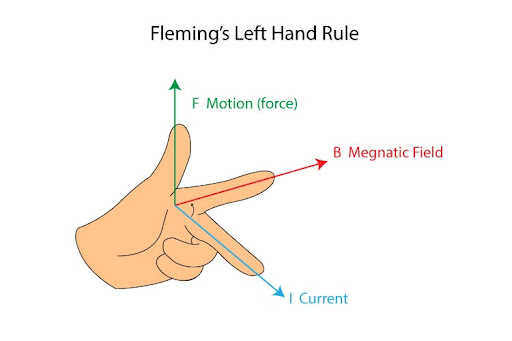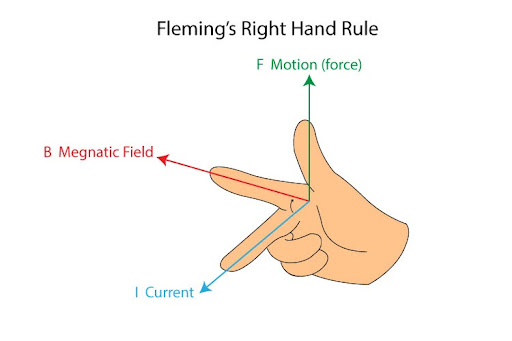# What is Fleming’s left hand rule?

The Fleming’s Left Hand Rule is a visual mnemonic - i.e. a visual aid that helps us understand how the directions of magnetic field, electric current and motion relate to one another in an electric motor. The principle behind an electric motor involves an electric current placed in an external magnetic field, which in turn generates a magnetic force that acts on the current-carrying conductor itself (usually in the form of a coil).
Now, the interesting thing to note here is this: the directions of this magnetic field, current and force (that creates motion) are all mutually perpendicular. So the use of the thumb, first and second fingers really helps us arrange the three directions in such a way that they all point at right angles. That can be done as follows:
Close your left hand in a fist, and raise it forwards such that the back of your hand points to the left. Give a thumbs up (literally!), point your first finger forwards, and then direct your second finger sharply towards the right. Your fingers should now be arranged in the following manner:A good way of memorizing who’s what is this:
The ThuMb represents the Motion (caused by the force)
The First Finger represents the Magnetic Field
The SeCond Finger represents the Current
Keep in mind that the current type we’re following is the conventional current - i.e. we assume the current moves from positive to negative terminals.

Warning: this rule only applies with the Left Hand, because Fleming’s Right Hand Rule serves a completely different purpose.

The Fleming’s Right Hand Rule applies to electric generators - which run on the fundamentals of electromagnetic induction, wherein a conducting coil is made to rotate within an external magnetic field to produce an electric current. It thus applies to the right hand, and it looks like so: